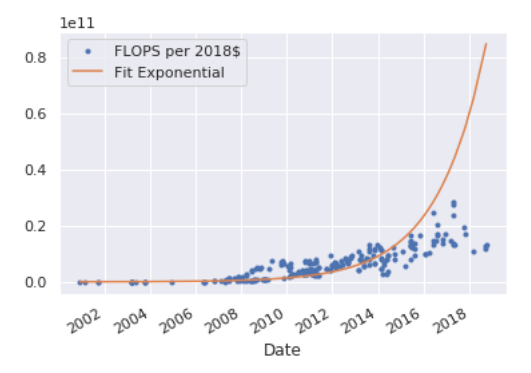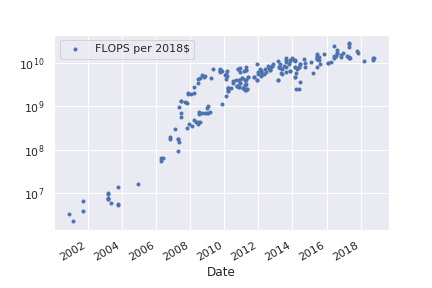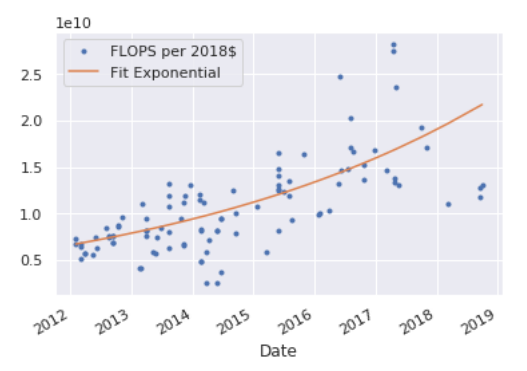# Introduction

Many of the impressive results in deep learning in recent years have been achieved through massive investment in hardware needed for training, with projects like AlphaGo Zero using \$25 million worth of computer hardware. Given this, improvements in price-performance of hardware used for deep learning will play an important role in determining the scale of projects in the coming years.

While machine learning ASICs like TPUs are likely the future, the recent deep learning boom was powered by GPUs1. The architectures of TPUs and GPUs differ in important ways, but much of the design and fabrication process is similar and both are largely focused on efficient, parallelized arithmetic2, so trends observed in GPUs can inform us about what to expect from TPUs.

Commonly mentioned figures for the price-performance generalization of Moore’s Law suggest that price-performance doubles roughly every two years, but this figure warranted further investigation.

# Data

We constructed a data set containing the model name, launch date, single precision performance in GFLOPS, and release price in non-inflation adjusted US dollars for 223 Nvidia and AMD GPUs (scraped from Wikipedia)3. The data set covered almost two decades, so prices were adjusted to 2018 US dollars using the Consumer Price Index.

# Analysis

Fitting an exponential to the data-set yielded the curve (where t equals the number of years since the start of 2070):

\begin{equation} f(t) \approx 2.26 e^{0.470 t} \end{equation}This implies a doubling time of ~1.5 years. It should be noted that this is somewhat misleading because the price-performance curve isn’t a clean exponential. Inspecting a log-plot suggests that price-performance has been in a distinctly slower growth regime since around 2012.Fitting to data from 2012 or later yields the curve:

\begin{equation} f(t) \approx 15.2 e^{0.176 t}, \end{equation}

corresponding to a doubling time of ~3.9 years.# External Discussion

1. GPUs are still more cost effective than TPUs, but have lower serial computation speed.

2. This is not nearly as true as with CPUs which have managed to extract performance improvements from increasingly arcane changes to control circuitry

3. AI Impacts has a similar data set